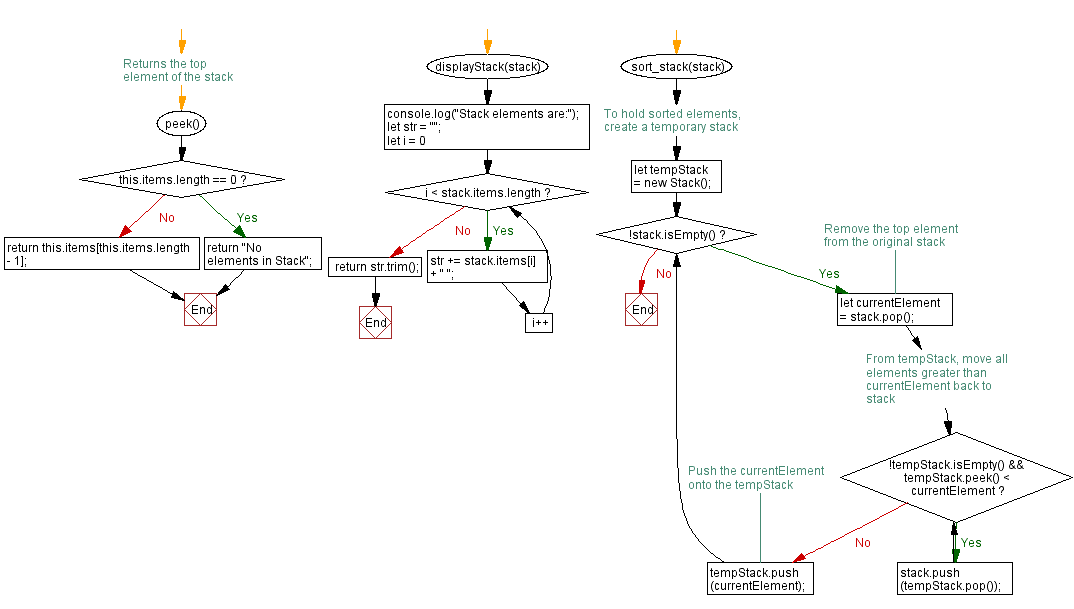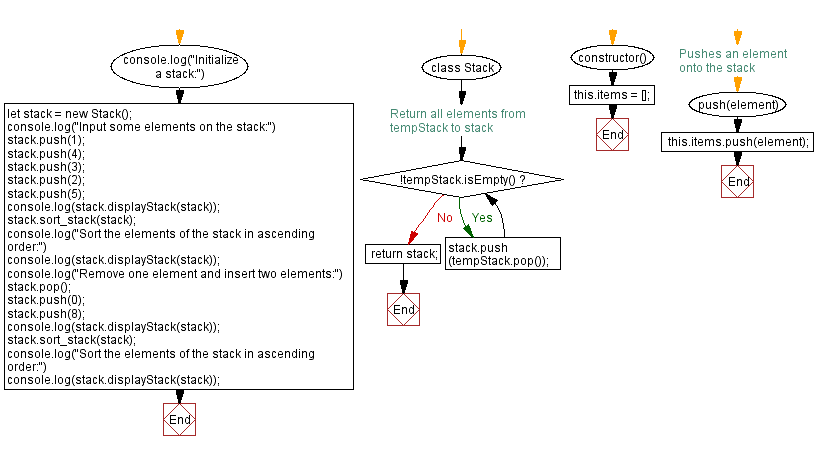# JavaScript Exercises: Sort the elements of a stack in ascending order

## JavaScript Stack: Exercise-3 with Solution

Write a JavaScript program to sort the elements of a given stack in ascending order.

Sample Solution:

JavaScript Code:

``````class Stack {
constructor() {
this.items = [];
}

// Pushes an element onto the stack
push(element) {
this.items.push(element);
}

// Remove the top element from the stack and return it
pop() {
if (this.items.length == 0)
return "Underflow";
return this.items.pop();
}

// Checks if the stack is empty
isEmpty() {
return this.items.length == 0;
}

// Returns the top element of the stack
peek() {
if (this.items.length == 0)
return "No elements in Stack";
return this.items[this.items.length - 1];
}

displayStack(stack) {
console.log("Stack elements are:");
let str = "";
for (let i = 0; i < stack.items.length; i++)
str += stack.items[i] + " ";
return str.trim();
}
sort_stack(stack) {
// To hold sorted elements, create a temporary stack
let tempStack = new Stack();
while (!stack.isEmpty()) {
// Remove the top element from the original stack
let currentElement = stack.pop();
// From tempStack, move all elements greater than currentElement back to stack
while (!tempStack.isEmpty() && tempStack.peek() < currentElement) {
stack.push(tempStack.pop());
}
// Push the currentElement onto the tempStack
tempStack.push(currentElement);
}
// Return all elements from tempStack to stack
while (!tempStack.isEmpty()) {
stack.push(tempStack.pop());
}
return stack;
}
}
console.log("Initialize a stack:")
let stack = new Stack();
console.log("Input some elements on the stack:")
stack.push(1);
stack.push(4);
stack.push(3);
stack.push(2);
stack.push(5);
console.log(stack.displayStack(stack));
stack.sort_stack(stack);
console.log("Sort the elements of the stack in ascending order:")
console.log(stack.displayStack(stack));
console.log("Remove one element and insert two elements:")
stack.pop();
stack.push(0);
stack.push(8);
console.log(stack.displayStack(stack));
stack.sort_stack(stack);
console.log("Sort the elements of the stack in ascending order:")
console.log(stack.displayStack(stack));
```
```

Sample Output:

```Initialize a stack:
Input some elements on the stack:
Stack elements are:
1 4 3 2 5
Sort the elements of the stack in ascending order:
Stack elements are:
1 2 3 4 5
Remove one element and insert two elements:
Stack elements are:
1 2 3 4 0 8
Sort the elements of the stack in ascending order:
Stack elements are:
0 1 2 3 4 8
```

Flowchart:Live Demo:

See the Pen javascript-stack-exercise-3 by w3resource (@w3resource) on CodePen.

Improve this sample solution and post your code through Disqus

Stack Previous: Sort the elements of a stack in descending order.
Stack Exercises Next: Reverse the elements of a stack.

What is the difficulty level of this exercise?

Test your Programming skills with w3resource's quiz.

﻿